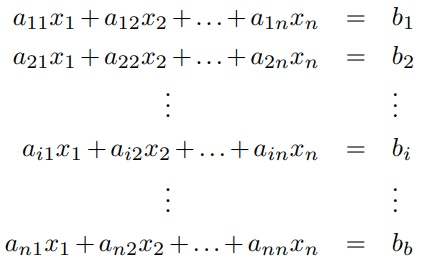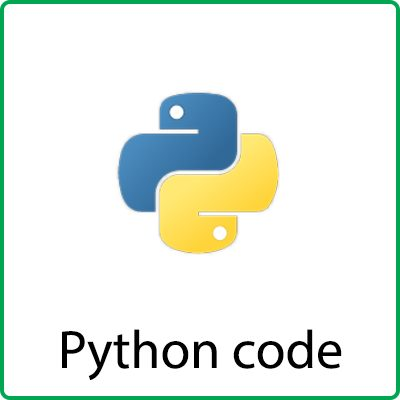# The Jacobi Method for linear system of equations

## MATLAB and Python code for Jacobi method :

Given the linear system of equations:From the above equation, follows that:The Jacobi method is an iterative method, which starts from an initial guess for the solutionThen, the solution in iteration k is used to find an approximation for the system solution in iteration k + 1. This is done as follows:Generally, the solution in iterationcan be written in the form:The Jacobi iteration stops when,for some arbitraryExample 2.1 Write the first three iterations of the Jacobi method, for the linear system:starting from the zeros vectorSolution:

We write:1. First iteration k = 0:2. Second iteration k = 1:3. Third iteration k = 2:Example 2.2 The Jacobi method will be applied for solving the linear system:## MATLAB code :

```function x = JacobiSolve(A, b, Eps)
n = length(b) ;
x0 = zeros(3, 1) ;
x = ones(size(x0)) ;
while norm(x-x0, inf) >= Eps
x0 = x ;
for i = 1 : n
x(i) = b(i) ;
for j = 1 : n
if j ~= i
x(i) = x(i) - A(i, j)*x0(j) ;
end
end
x(i) = x(i) / A(i, i) ;
end
end```

## Python code :## Reviews

There are no reviews yet.

Categories: ,